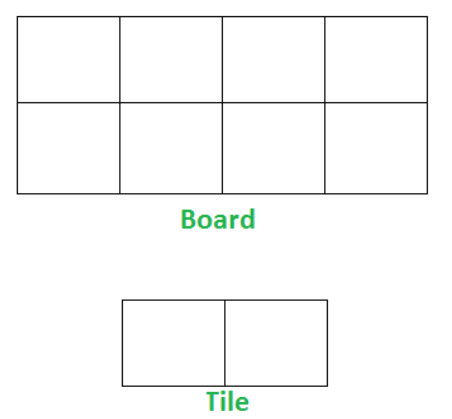New update is available. Click here to update.
Last Updated: 25 Jul, 2020
##### Tiling Problem
Hard
Problem statement#### You have been given a board where there are '2' rows and 'N' columns. You have an infinite supply of 2x1 tiles, and you can place a tile in the following ways:

``````1. Horizontally as 1x2 tile
2. Vertically as 2x1 tile
``````

#### Count the number of ways to tile the given board using the available tiles.

##### Note :
``````The number of ways might be large so output your answer modulo 10^9 + 7.
``````

#### Here an example of tile and board for 'N' = 4 :##### Input format :
``````The first and only line of each test case contains an Integer 'N' which denotes the size of the board, i.e. '2' rows and 'N' columns.
``````
##### Output format :
``````For each test case, print the number of ways to tile the board modulo 10^9 + 7.
``````
##### Note:
``````You are not required to print the output explicitly, it has already been taken care of. Just implement the function.
``````
##### Constraints :
``````1 <= N <= 10^18

Where 'N' is the number of columns in the board.

Time limit: 1 sec
``````Approaches

## 01ApproachTry to place the tile to fill the unit column and calculate the number of ways from smaller sub-problems. Then use memoization to convert O(2^N) solution to an O(N) solution.

1. At any point we are at ‘idx’ column then we can place our tile in two ways to fill this column.
1. Option 1 -  1 Horizontal Tile

We can place in this way where we have ‘idx-1’ column filled.

2.   Option 2 - 2 Vertical Tiles

We can place in this way where we have ‘idx-2’ column filled.

2. So, numberOfWays(n) = numberOfWays(n-1) + numberOfWays(n-2)

3. Base cases are:

1. When n = 1 there is only 1 way - Placing 1 Vertical Tile
2. When n = 2 there are two ways - Placing 2 Vertical Tile and Placing 2 Horizontal Tiles.
3. Also, take care of overflow using modulo 10^9 + 7.
4. Lastly, use a DP Array of size N for memoization to save time over repetitive calls.